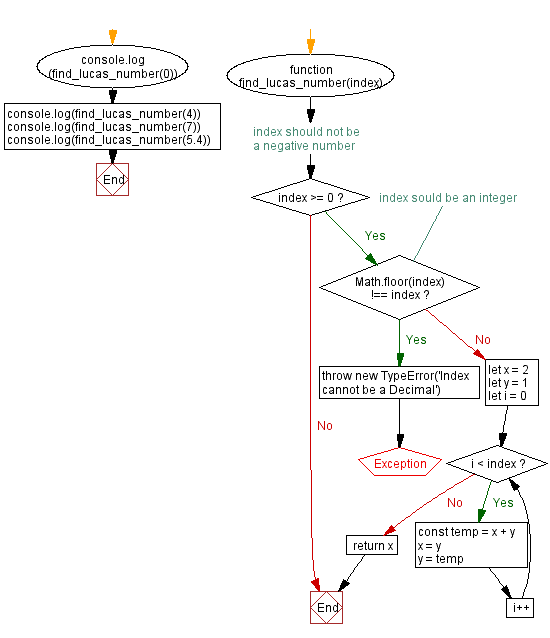# JavaScript: Find Lucas number from index value

## JavaScript Math: Exercise-65 with Solution

Write a JavaScript program to get the Nth Lucas Number.

From Wikipedia-
The Lucas numbers or Lucas series are an integer sequence named after the mathematician Francois Edouard Anatole Lucas (1842-1891), who studied both that sequence and the closely related Fibonacci numbers. Lucas numbers and Fibonacci numbers form complementary instances of Lucas sequences.
The Lucas series has the same recursive relationship as the Fibonacci sequence, where each term is the sum of the two previous terms, but with different starting values. This produces a sequence where the ratios of successive terms approach the golden ratio, and in fact the terms themselves are roundings of integer powers of the golden ratio. The sequence also has a variety of relationships with the Fibonacci numbers, like the fact that adding any two Fibonacci numbers two terms apart in the Fibonacci sequence results in the Lucas number in between.
The first few Lucas numbers are:
2, 1, 3, 4, 7, 11, 18, 29, 47, 76, 123, 199, 322, 521, 843, 1364, 2207, 3571, 5778, 9349 .... .

Sample Data:
0 -> 2
4 -> 7
7 -> 29

Sample Solution:

HTML Code:

``````<!DOCTYPE html>
<html>
<meta charset="utf-8">
<title>JavaScript function to find Lucas number from index value</title>
<body>

</body>
</html>
```
```

JavaScript Code:

``````function find_lucas_number(index) {
// index should not be a negative number
if (index >= 0)
{
// index sould be an integer
if (Math.floor(index) !== index) throw new TypeError('Index cannot be a Decimal')
let x = 2
let y = 1
for (let i = 0; i < index; i++) {
const temp = x + y
x = y
y = temp
}
return x
}
}
console.log(find_lucas_number(0))
console.log(find_lucas_number(4))
console.log(find_lucas_number(7))
console.log(find_lucas_number(5.4))
```
```

Sample Output:

```2
7
29
--------------------------------------------------
Uncaught TypeError: Index cannot be a Decimal
at https://cdpn.io/cpe/boomboom/pen.js?key=pen.js-fb7a2744-ca9f-8c12-e963-385a69e41cff:6
```

Flowchart:Live Demo:

See the Pen javascript-math-exercise-65 by w3resource (@w3resource) on CodePen.

Improve this sample solution and post your code through Disqus

Previous: Volume of a Hemisphere.
Next: Twin prime of a prime number

What is the difficulty level of this exercise?

Test your Programming skills with w3resource's quiz.

﻿